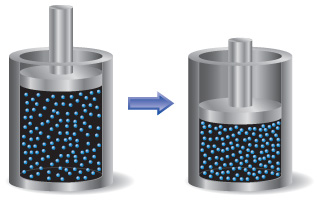# Problem: A sample of gas is contained in a cylinder-and-piston arrangement. It undergoes the change in state shown in the drawing. Assume first that the cylinder and piston are perfect thermal insulators that do not allow heat to be transferred.Now assume that the cylinder and piston are made up of a thermal conductor such as a metal. During the state change, the cylinder gets warmer to the touch. What is the sign of  q  for the state change in this case? Describe the difference in the state of the system at the end of the process in the two cases. What can you say about the relative values of E?

###### FREE Expert Solution

ΔE = q + w

First system:

thermal insulator → q =0

piston compress gas → +w

99% (2 ratings)###### Problem Details

A sample of gas is contained in a cylinder-and-piston arrangement. It undergoes the change in state shown in the drawing. Assume first that the cylinder and piston are perfect thermal insulators that do not allow heat to be transferred.Now assume that the cylinder and piston are made up of a thermal conductor such as a metal. During the state change, the cylinder gets warmer to the touch. What is the sign of  q  for the state change in this case? Describe the difference in the state of the system at the end of the process in the two cases. What can you say about the relative values of E?